# Drawing in a Mirror – Mirror Letters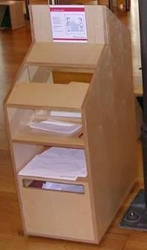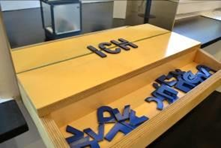Drawing in a mirror: Look into the mirror and try to trace the figure on the sheet or write your name! Why is that difficult? Mirror Letters: Place the half-letters next to the mirror to complete them. What words can you make from the mirror letters? Which letters have horizontal symmetry? Which letters have vertical symmetry? Which letters have no lines of symmetry?
Maths

Mirrors show lengths and angles as they originally are, but they do reverse depth. That’s why drawing in a mirror can be so difficult. Objects that are furthest away from us (and closest to the mirror) appear closest to us. And objects close to us (and furthest from the mirror) appear furthest away in the mirror.A shape has mirror symmetry if there is a line that divides the shape into two halves that are exact mirror images of each other.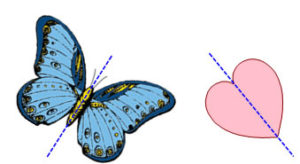A horizontal line of symmetry means the top half is a reflection of the bottom half. A vertical line of symmetry means the left half is a reflection of the right half.

Some letters have horizontal symmetry, some letters have vertical symmetry, some letters have both, and some letters have no mirror symmetry at all.You can find if a shape has a line of symmetry by folding it. If the shape folds perfectly in half (all edges matching), then the shape has a line of symmetry. For example, a rectangle has a horizontal line of symmetry and a vertical line of symmetry.But a rectangle does not always have a diagonal line of symmetry. The two halves do not match.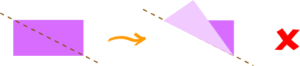History

The first mirrors were probably pools of water. About 2000 years ago people started using glass, coated with metal. These mirrors were smooth and highly reflective. Classical mathematicians were interested in reflections because they preserve the shape of objects, in other words the shape looks the same before and after the reflection. Other actions that preserve shapes include rotations and translations (moving the shape across).
Later, mathematicians realised that they can apply the same ideas to other things we want to stay the same. This might be shape, length, angle, volume, or number. In physics, we can apply the same ideas to things like energy or momentum.

People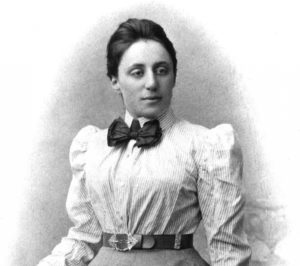Emmy Noether 1882 – 1935 Emmy Noether was a German mathematician. Noether studied the mathematics of symmetry and applied those ideas to physics, where they become conservation laws. It is considered one of the most important results in modern physics.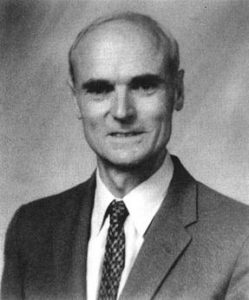Donald Coxeter 1917 – 2003 Donald Coxeter was a British-born Canadian mathematician. Coxeter studied shapes and symmetry, especially symmetry made by repeated reflections. Coxeter also wrote about the connection between maths and music, as well as books about mathematics for young people.
Applications

Mirrors are used in many technologies such as camera, televisions, microscopes and telescopes. Sometimes this involved reflecting an image several times in different mirrors.
In mathematics, symmetry means there is something important you want to keep the same. This might be shape, length, angle, volume or number. In physics, it is things like energy or momentum that we want to stay the same, these are the conservation laws of physics. In chemistry, the shape and symmetry of molecules can affect how the chemistry behaves

Maths at Home

What are these mirror calculations?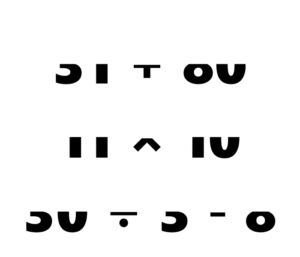Can you make up some other calculations that have horizontal symmetry?

Can you make up a calculation where the calculation and the both have horizontal symmetry?